Question

# (a) Place the following gases in order of increasing average molecular speed at 25°C: CO, SF6,...

(a) Place the following gases in order of increasing average molecular speed at 25°C: CO, SF6, H2S, Cl2, HI. (Type your answer using the format SF6 for SF6.) slowest < < < < fastest (b) Calculate the rms speed of CO at 45.0°C. m/s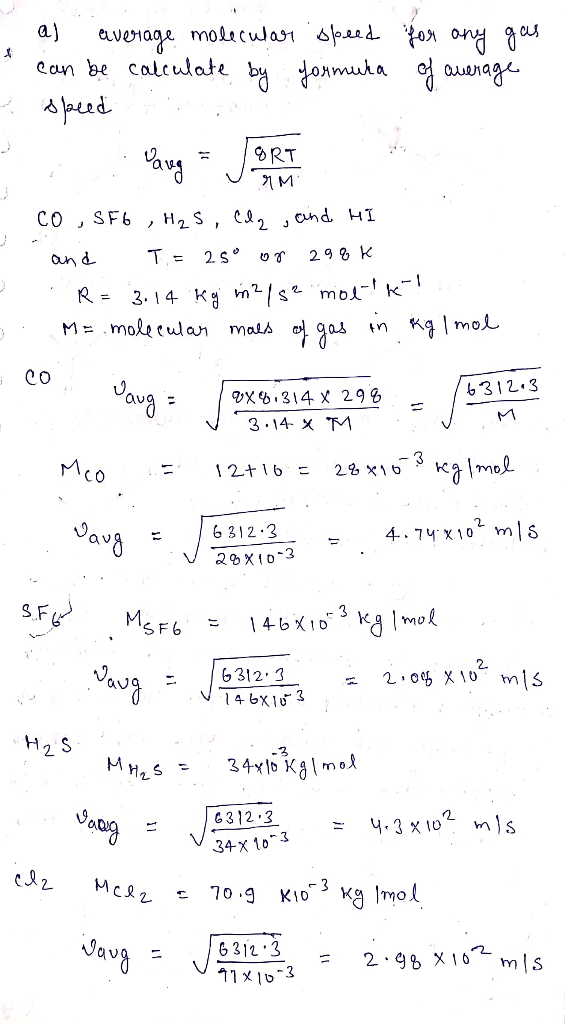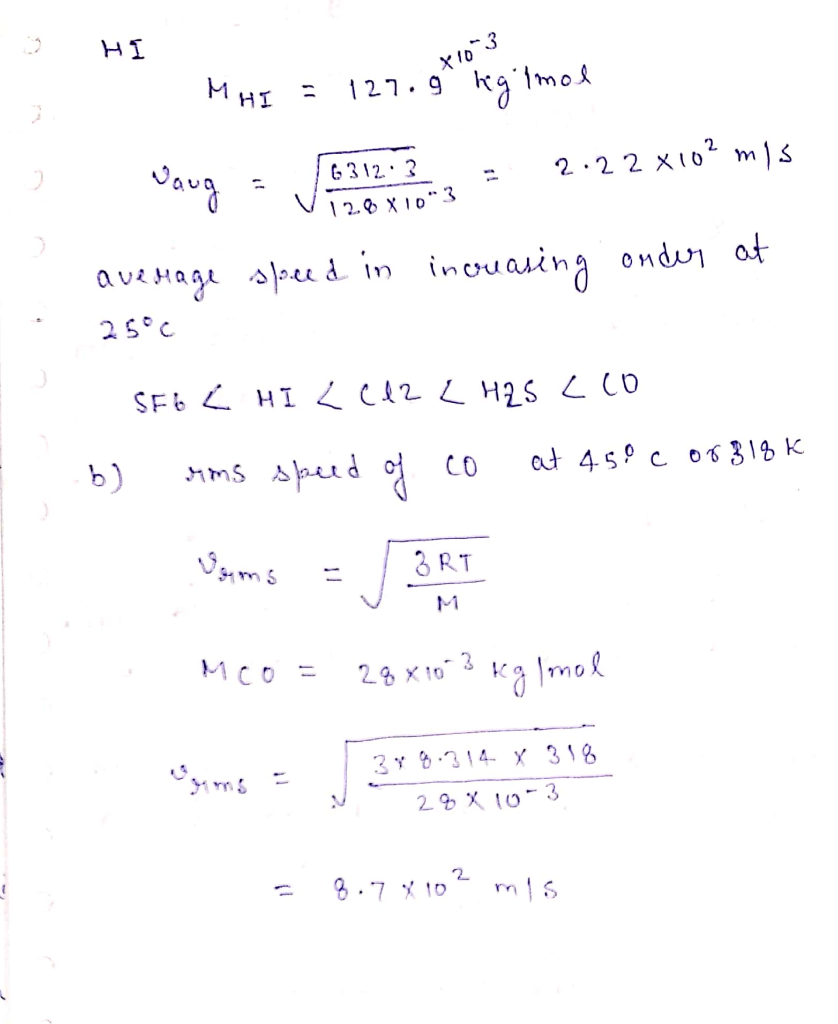#### Earn Coins

Coins can be redeemed for fabulous gifts.

Similar Homework Help Questions
• ### ) Place the following gases in order of increasing average molecular speed at 25°C: CO, SF6,...

) Place the following gases in order of increasing average molecular speed at 25°C: CO, SF6, H2S, Cl2, HI. slowest < < < < fastest (b) Calculate the rms speed of CO at 65.0°C. m/s

• ### (a) Place the following gases in order of increasing average molecular speed at 25 C: CO,...

(a) Place the following gases in order of increasing average molecular speed at 25 C: CO, SF6, H2S, Cl2, HI (b) calculate the rms speed of CO at 70.0 C

• ### Arrange the following molecules in order of increasing average molecular speed. All are at the same...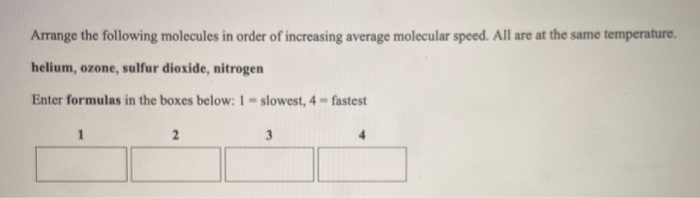Arrange the following molecules in order of increasing average molecular speed. All are at the same temperature, helium, ozone, sulfur dioxide, nitrogen Enter formulas in the boxes below: 1 - slowest, 4 - fastest

• ### Arrange the following molecules in order of increasing average molecular speed. All are at the same...

Arrange the following molecules in order of increasing average molecular speed. All are at the same temperature. carbon dioxide, hydrogen, krypton, oxygen Enter formulas in the boxes below: 1 = slowest, 4 = fastest 1 2 3 4

• ### Arrange the following molecules in order of increasing average molecular speed. Ar at 387K, CHA at...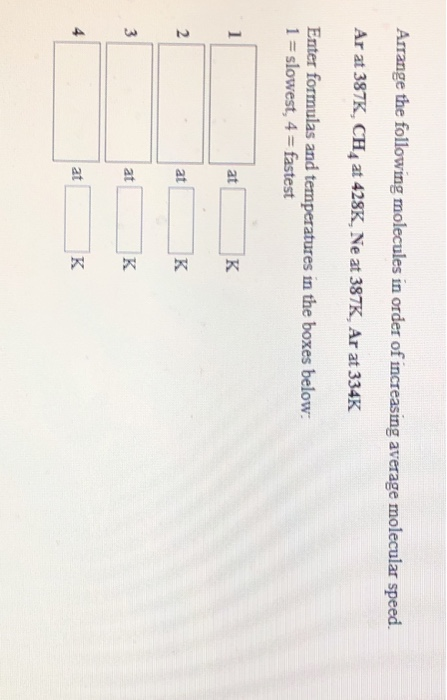Arrange the following molecules in order of increasing average molecular speed. Ar at 387K, CHA at 428K, Ne at 387K, Ar at 334K Enter formulas and temperatures in the boxes below: 1 = slowest, 4 = fastest

• ### Arrange the following gases in order of increasing average molecular speed at 300 K. F2 HF...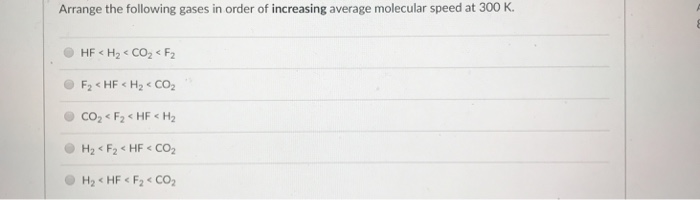Arrange the following gases in order of increasing average molecular speed at 300 K. F2 HF H2 CO2 H2< F2HF CO2

• ### Arrange the following molecules in order of increasing average molecular spee H2 at 451K, Ar at...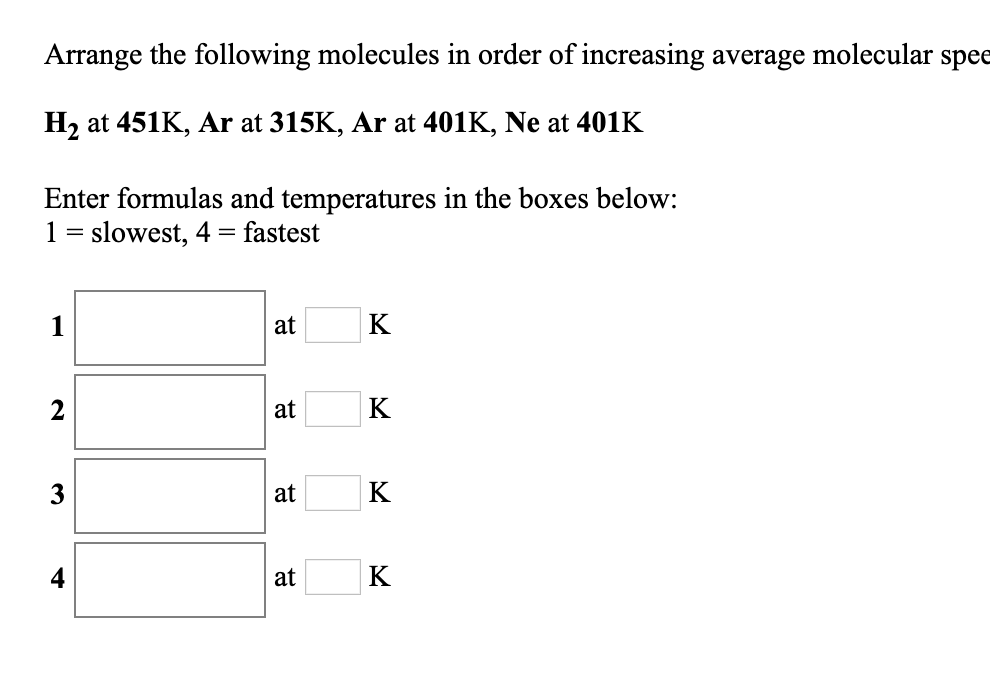Arrange the following molecules in order of increasing average molecular spee H2 at 451K, Ar at 315K, Ar at 401K, Ne at 401K Enter formulas and temperatures in the boxes below: 1 slowest, 4 = fastest K 1 at K 2 at K 3 at K at Arrange the following molecules in order of increasing average molecular speed. All are at the same temperature. hydrogen, ozone, oxygen, krypton Enter formulas in the boxes below: 1 = slowest, 4 fastest 1...

• ### Arrange the following molecules in order of increasing average molecular speed. H2 at 431K, CO2 at...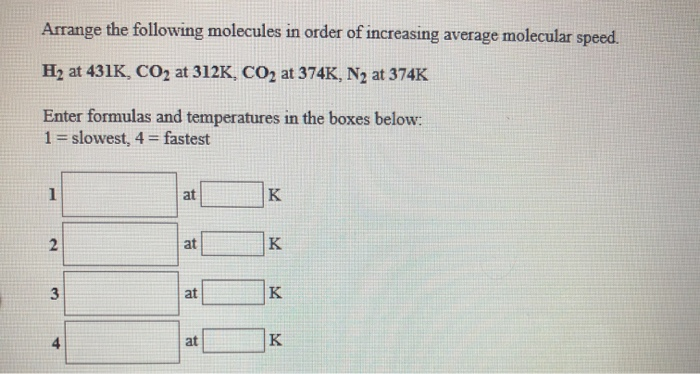Arrange the following molecules in order of increasing average molecular speed. H2 at 431K, CO2 at 312K, CO2 at 374K, N2 at 374K Enter formulas and temperatures in the boxes below: 1 slowest, 4 fastest K 1 at K 2 at K 3 at K at Match the gas sample on the left with a description on the right. You may use the same answer more than once. Clear All 0.299 moles of O3 in a 4.22 L container at...

• ### For the gas N2 calculate the volume in mL if 189 g were under a pressure...

For the gas N2 calculate the volume in mL if 189 g were under a pressure of 543 torr at 40.0 oC. A sample of gas contains 62.0 moles at 327 K in 4.00 L of volume. What is the pressure (in atm) of the gas? What is the density (in mg/mL) of a H2S gas at 12.9 atm and 137 K Place the following gases in order of increasing average molecular speed at 25 oC: Slowest → Fastest energy...

• ### Q9. Which of the following gases has the slowest Root-Mean-Square Speed (rms) at a temperature of...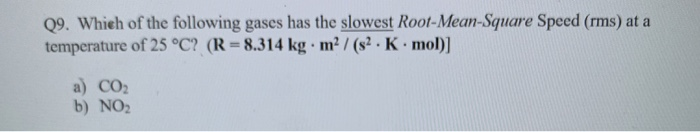Q9. Which of the following gases has the slowest Root-Mean-Square Speed (rms) at a temperature of 25 °C? (R = 8.314 kg. m2 / (52. K.mol)] a) CO2 b) NO2 c) H2S d) SO2 e) Cl2